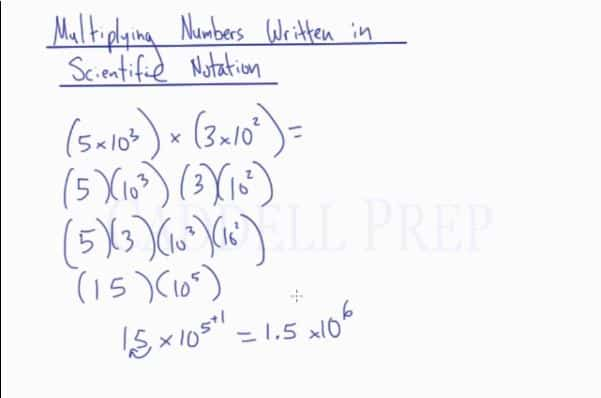## Examples of Operations with Scientific Notation

### Example 1$(9\times 10^4) \times (6 \times 10^8)$

First, let’s break it down to$(9) \times (10^4) \times (6) \times (10^8)$

Next, group the like terms then multiply$(9) \times (6) \times (10^4) \times (10^8)$

We’ll have$54 \times 10^{12}$

Remember, it should be greater than or equal to$1$ and less than$10$.

So let’s simplify it further by moving one decimal space to the left.

We now have$5.4 \times 10^{13}$

### Example 2$\dfrac{36 \times 10^9}{6 \times 10^5}$

Let’s divide the like terms$36 \div 6$ is$6$$10^9 \div 10^5$ is$10^4$

So our answer is$6 \times 10^4$

## Video-Lesson Transcript

Let’s multiply and divide numbers written in scientific notation. After you finish this lesson, view all of our Pre-Algebra lessons and practice problems.

Now, let’s move on to multiplying numbers written in scientific notation.

Here’s an example$(5 \times 10^3) \times (3 \times 10^2)$

Everything here should be multiplied

Let’s break it down to$(5) \times (10^3) \times (3) \times (10^2)$

Let’s group the like terms first then multiply$(5) \times (3) \times (10^3) \times (10^2)$

We’ll have$(15) \times (10^5)$$15 \times 10^5$ may look like a scientific notation but it’s not.

Remember,$a$ should be greater than or equal to$1$ and less than$10$.

So let’s simplify it further by moving one decimal space to the left.

We now have$1.5 \times 10^6$Let’s have two more examples.$(3.2 \times 10^4) \times (2 \times 10^7)$

Let’s multiply now$3.2 \times 2$ is$6.4$

then$10^4 \times 10^7$ is$10^11$

So now we have$6.4 \times 10^11$

We don’t have to make any adjustment because it is already in scientific notation form.

Let’s try this one$(5 \times 10^2) \times (9 \times 10^5)$$5 \times 9$ is$45$$10^2 \times 10^5$ is$10^7$

So we have$45 \times 10^7$

Then let’s move the decimal to get a value between$1$ and$10$.

The answer is$4.5 \times 10^8$

Now, let’s do division of numbers written in scientific notation.

For example:$\dfrac{8 \times 10^5}{2 \times 10^2}$

Let’s divide the like terms$8 \div 2$ is$4$$10^5 \div 10^2$ is$10^3$

So our answer is$4 \times 10^3$

Let’s have a more difficult one$\dfrac{3 \times 10^8}{6 \times 10^4}$

Let’s do this$3 \div 6$ is$0.5$$10^8 \div 10^4$ is$10^4$

We have$0.5 \times 10^4$

But this is not a scientific notation.

To write it down, we have to move the decimal space.

The final answer should be$5 \times 10^3$